Courses

# Quantum Numbers And Its Related Principles - Atomic Structure Chemistry Notes | EduRev

## Chemistry : Quantum Numbers And Its Related Principles - Atomic Structure Chemistry Notes | EduRev

The document Quantum Numbers And Its Related Principles - Atomic Structure Chemistry Notes | EduRev is a part of the Chemistry Course Physical Chemistry.
All you need of Chemistry at this link: Chemistry

Quantum Numbers

To understand the concept of Quantum Numbers, we must known the meaning of some terms clearly so as to avoid any confusion.

• Energy Level:  The non-radiating energy paths around the nucleus are called as Energy Levels, of Shells. These are specified by numbers having values 1, 2, 3, 4,…… or K, L, M, N,……… in order of increasing energies. The energy of a particular level is fixed.
• Sub-Energy Level:  The phenomenon of splitting of spectral lines in electric and magnetic fields reveals that there must be extra energy levels within a definite energy level. These were called as Sub-Energy Levels or Sub-Shells. There are four types of sub-shells namely; s, p, d, f.

First energy level (K or) has one sub-shell designated as 1s, the second energy level (L and 2) has two sub-shell as 2s & 2p, the third energy level (M or 3) has three sub shell as 3s, 3p and 3d, and the fourth energy level (N or 4) has four sub-shells as 4s, 4p, 4d and 4f. The energy of sub-shell increases roughly in the order: s < p < d < f.

• Orbital: Each sub-energy level (sub-shell) is composed of one or more orbitals. These orbitals belonging to a particular sub-shell have equal energies and are called as degenerate orbitals.
s-sub-shell has one orbital, p has three orbitals, d have five orbitals and f has seven orbitals.

To describe or to characterize the electrons around the nucleus in an atom, a set of four numbers is used, called as Quantum Numbers. These are specified such that the states available to the electrons should follow the laws of quantum mechanism or wave mechanics.

• Principal Quantum Number: (n): This quantum number represents the main energy levels (principal energy levels) designated as n = 1, 2, 3,………… or the corresponding shells are named as K, L, M, N,….. respectively. It gives an ideal of position and energy of an electron. The energy level n = 1 corresponds to minimum energy and subsequently n = 2, 3, 4,……, are arranged in order of increasing energy.

Higher is the value of n, greater is its distance from the nucleus, greater is its size and also greater is its energy. It also gives the total electrons that may be accommodated in each shell, the capacity of each shell is given by the formula 2n2, where n: principal quantum number.

• Azimuthal Quantum Number: (l): This number determines the energy associated with the angular momentum of the electron about the nucleus. It is also called as the angular momentum quantum number. It accounts for the appearance of groups of closely packed spectral lines in electric field.

It can assume all integral values from 0 to n –1. The possible values of l are:

0, 1, 2, 3, ……., n – 1

Each value of l describes a particular sub-shell in the main energy level and determines the shape of the electron cloud.

When n = 1, l = 0, i.e., its energy level contains one sub-shell which is called as a s-sub-shell. So for l = 0, the corresponding sub-shell is a s-sub-shell. Similarly when l = 1, 2, 3, the sub-shell are called p, d, f sub-shell respectively.

As you known for n = 1, l = 0, there is only one sub-shell. It is represented by 1s. Now for n = 2, l can take two values (the total number of values taken by l is equal to the value of n in a particular level). The possible values of l are 0, 1. The two sub-shell representing the IInd energy level are 2s, 2p. In the same manner, for n = 3, three sub-shell are designated as 3s, 3p, 3d corresponding to l = 0, 1, 2, and for n = 4, four sub-shells are designated as 4s, 4p, 4d, 4f corresponding to l = 0, 1, 2, 3, .

The orbital Angular momentum of electron =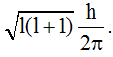• Magnetic Quantum Number (m):  An electron with angular momentum can be thought as an electric current circulating in a loop. A magnetic field due to this current is observed. This induced magnetism is determined by the magnetic quantum number. Under the influence of magnetic field, the electrons in a given sub-energy level prefer to orient themselves in certain specific regions in space around the nucleus. The number of possible orientations for a sub-energy level is determined by possible values of m corresponds to the number of orbitals in a given sub-energy level).

m can be have any integral values between –l to + l including 0, i.e., m = –l, 0+, l, ……0, 1, 2, 3, 4,……….l, –1, +l.

We can say that a total of (2l + 1) values of m are there for a given value of l–2, –1, 0, 1, 2, 3.

In s sub-shell there is only one orbital [l = 0, → m = (2l + 1) = 1].

In p sub-shell there are three orbitals corresponding to three values of m: –1, 0 + 1. [l = 1 → m = (2l + 1) = 3]

These three orbitals are represented as px, py, pz along X, Y, Z axes perpendicular to each other.

In d sub-shell, there are five orbitals corresponding to –2, –1, +2, [l = 2, → m = (2 × 2 + 1) = 5]. These five orbitals are represented as dxy, dzx, .

In f sub-shell there are seven orbitals corresponding to –3, –2, –1, 0, +1, +2, +3, [l = 3 → m = (2×3 + 1) = 7]

• Spin quantum Number (s): When an electron rotates around a nucleus it also spins about axis. If the spin is clockwise, its spin quantum number if +1/2 and is represented as ↑. If the spin is anti-clockwise, its value is –1/2 and is represented as ↓. If the value of s is +1/2, then by convention, we take that electron as the first electron in that orbital and if the value of s is –1/2, it is taken as second electron.

Aufbau Principle:

Aufbau is a German word meaning ‘building up’. This gives us a sequence in which various sub-shells are filled up depending on the relative order of the energy of the subs-hells. The sub-shell with minimum energy is filled up first and when this obtains maximum quota of electrons, then the next sub-shell of higher energy starts filling.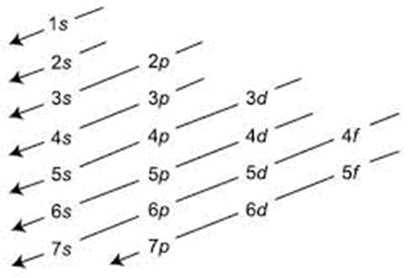Exceptions of Aufbau Principle:  In some cases it is seen that actual electronic arrangement is slightly different from arrangement given by Aufbau principle. A simple reason behind this is that half-filled and full-filled sub-shell have got extra stability.

Cr(24)  → 1s2, 2s22p6, 3s23p63d4, 4s2              (Wrong)

→ 1s2, 2s22p6, 3s23p63d5, 4s1                          (Right)

Cu(29) → 1s2, 2s22p6, 3s23p63d9, 4s2              (Wrong)

→ 1s2, 2s22p6, 3s23p63d10, 4s1                         (Right)

Similarly the following elements have slightly different configurations than expected.

Nb → [Kr] 4d45s1

No → [Kr] 4d45s1

Ru → [Kr] 4d75s1

Ph → [Kr] 4d85s1

Pd → [Kr] 4d105s0

Ag → [Kr] 4d105s1

Pt → [Xe] 4d145s96s1

Au → [Xe] 4d145d106s1

Shapes of Atomic Orbitals

•  S-orbital: An electron in considered to be immersed out in the form of a cloud. The shape of the cloud is the shape of the orbital. The cloud is not uniform but denser is the region where the probability of finding the electron in maximum.

The orbital with the lowest energy is the 1s orbital. It is a sphere with its center of the nucleus of the atom. The s-orbital is said to spherically symmetrical about the nucleus, so that the electronic charge is not concentrated in any particular direction. 2s orbital is also spherically symmetrical about the nucleus, but it is larger than (i.e., away from) the 1s orbit.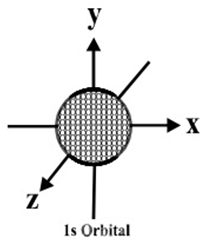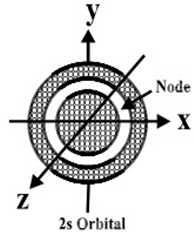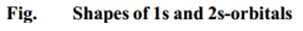• p-orbitals: There are three p-orbitals: px, py and pz. they are dumb-bell shaped, the two levels being separated by; a nodal plane, i.e., a plane where there is no likely hood of finding the electron. The p-orbitals have a marked direction character, depending as whether px, py and pz orbital is being considered. The p-orbitals consist of two lobes with the atomic nucleus lying between them. The axis of each p-orbitals is perpendicular to the other two. The px, py and pz orbitals are equivalent except for their directional property. They have same energy; orbitals having the same energy are said to be degenerated.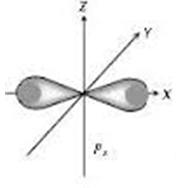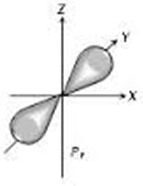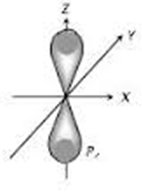• d-orbitals: There are d-orbitals. The shapes of four d-orbitals resemble four leaf cloves. The fifth d-orbital loops different. The shapes of these orbitals are given below.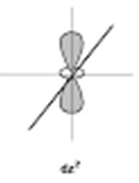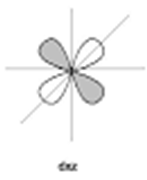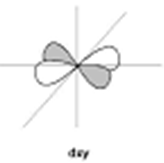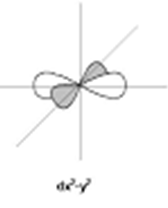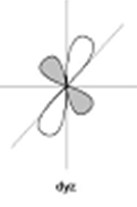Dual Character

In case of light some phenomenon like diffraction and interference can be explained on the basis of its wave character. However, the certain other phenomenon such as black body radiation and photoelectric effect can be explained only on the basis of its particles nature. Thus, light is said to have a dual character. Such studies on light were made by Einstein in 1905.

Louis de-Broglie, in 1942 extended the ideal of photons to material particles such as electron and he proposed that matter also has a dual character-as wave and as particle.

Derivation of de-Broglie equation: The wavelength of the wave associated with any material particle was calculated by analogy with photon, if it is assumed to have wave character, its energy is given by

E = hν ……….(i) (according to the Planck’s quantum theory)

Where ν is the frequency of the wave and ‘h’ is Plack’s constant

If the photon is supposed to have particle character, its energy is given by

E = mc2…….(ii) according to Einstein’s equation)

Where ‘m’ is the mass of photon, ‘c’ is the velocity of light.

By equating (i) and (ii)

hν = mc2

But ν = c/λ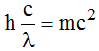Or λ = h/mc

The above equation is applicable to material particle if the mass and velocity of photon is replaced by the mass and velocity of material particle. Thus for any particle like electron.

λ = h/mv (or)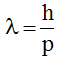Where mv = p is the momentum of the particle.

Heisenberg’s Uncertainty Principle:

All moving objects that we see around us e.g., a car, a ball thrown in the air etc, move along definite paths. Hence their position and velocity can be measured accurately at any instant of time. Is it possible for subatomic particle also:

As a consequence of dual nature of matter. Heisenberg, in 1927 gave a principle about the uncertainties in simultaneous measurement of position and momentum (mass × velocity) of small particles. This principle states.

It is impossible to measure simultaneously the position and momentum of a small microscopic moving particle with absolute accuracy or certainty i.e., if an attempt is made to measure any one of these two quantities with higher accuracy, the other becomes less accurate.

The product of the uncertainty in position (Δx) and the uncertainty in the momentum (Δp = m.Δv where m is the mass of the particle and Δv is the uncertainty in velocity) is equal to or greater than h/4p where h is the Planck’s constant.

Thus, the mathematical expression for the Heisenberg’s uncertainty principle is simply written as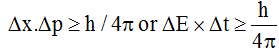Explanation of Heisenberg’s uncertainty Principle: Suppose we attempt to measure both the position and momentum of an electron, to pin point the position of the electron we have to use light so that the photon of light strikes the electron and the reflected photon is seen in the microscope. As a result of the hitting. The position as well as the velocity of the electron  is distributed. The accuracy with which the position of the particle can be measured depends upon the wavelength of the light used. The uncertainty in position is +l. The shorter the wavelength, the greater is the accuracy. But shorter wavelength means higher frequency and hence higher energy. This high energy photon on striking the electron changes its speed as well as direction. But this is not true for macroscopic moving particle. Hence Heisenberg’s uncertainty principle is not applicable to macroscopic particles.

Pauli’s Exclusion Principle: According to this principle

No two electrons in an atom can have the same set of all the quantum numbers or one can say that no two electrons can have the same quantized states.

Consider an electronic arrangement in 1st energy level (n = 1). For n = 1, l = 0, and m = 0. Now s can have to values corresponding to each value of m i.e., s = +1.2, –1/2 (n, 1, possible designation of an electron in a state with n = 1 is 1, 0, 0, +1/2 and 1, 0, 0, –1/2 (n, l, m, s) i.e., two quantized states. This implies that an orbital can accommodate (for n = 1, m = 0, → one orbital) maximum of two electrons having opposite spins.

The maximum number of electrons in the different sub-shells = 2 (2l + 1)

s-sub-shell = 2, p-sub-shell = 6, d-sub-shell = 10 and f-sub-shell = 14

Hund’s Rule of Maximum Multiplicity

• According to this rule: “Electrons never pair until no available empty degenerate orbitals are left to him”.

This means an electron always occupies a vacant orbital in the same sub-shell (degenerate orbital) and pairing starts only when all of the degenerate orbitals are filled up. This means that the pairing starts with 2nd electron in a sub-shell, 4th electron is p-sub-shell, 6th electron in d-sub-shell and 8th electron in f-sub-shell.

By doing this, the electrons stay as far away from each other as possible. This is highly reasonable if we consider the electron-electron repulsion. Hence electron obey Hund’s rule as it results in lower energy state and hence more stability.

Node and Nodal Plane

Node is defined as a region where the probability of finding an electron is zero.

The planes passing through the angular nodal points are called nodal planes.

Nodes

No. of radial or spherical nodes = n – l – 1

No. of angular nodes,= l, Total number of nodes = n – 1

Offer running on EduRev: Apply code STAYHOME200 to get INR 200 off on our premium plan EduRev Infinity!

## Physical Chemistry

66 videos|94 docs|32 tests

,

,

,

,

,

,

,

,

,

,

,

,

,

,

,

,

,

,

,

,

,

;